﻿ On the Existence of the Solution for q-Caputo Fractional Boundary Value ProblemPublications are Open
Access in this journal
Article Versions
Export Article
• Normal Style
• MLA Style
• APA Style
• Chicago Style
Research Article
Open Access Peer-reviewed

### On the Existence of the Solution for q-Caputo Fractional Boundary Value Problem

Norodin A. Rangaig , Caironesa T. Pada, Vernie C. Convicto
Applied Mathematics and Physics. 2017, 5(3), 99-102. DOI: 10.12691/amp-5-3-4
Published online: December 18, 2017

### Abstract

In this paper, we discussed the existence of a four point boundary value problem for q-fractional differential equation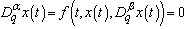in a Banach space, particulary, using the Banach contraction principle for certain conditions on f.

### 1. Introduction

Fractional calculus has been always an attractive field for many researchers due to its very wide applications in the study of modeling for various fields in science and engineering, such as physics, biophysics, chemistry, controlling engineering, visco-elasticity and many more. For further details, see 1, 2, 3, 4, 5 and the references therein. Years ago, the fractional equation has a been a significant progress (see 6, 7 and the references therein).

The q-calculus or known as "quantum"-calculus was originally developed by Jackson 8 and some of its basic definitions and properties can be found in 9.

This study focuses on showing the existence of the solution for the following problem: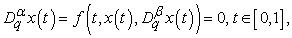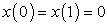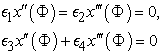(1)

where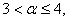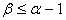and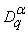and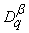are the q-Caputo fractional Derivatives,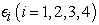are real constants and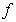is a continuous function on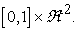This paper is organized as follows: in section 2, we introduce the prelimimaries of a q-analogue and definitions of q-fractional integral and differential. In section 3, we give some hypotheses to prove the existence of solution and also using the Banach contraction priciple.

### 2. Mathematical Preliminaries

The following notations, properties, definitions, and preliminary facts will be utlized throughout this study. Let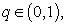then we have the q-analogue of a real number defined by 10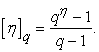The q-analogue of the power function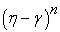with n is a nonnegative integer is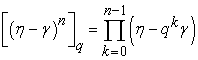in general, if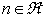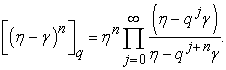The q-derivative of a function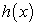is defined by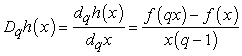and the q-integral of a functionin the interval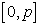is given by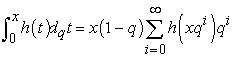The following an properties is useful in studying q-fractional calculus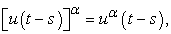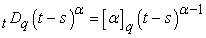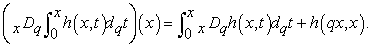Remark 2.1. It is to be noted that if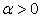and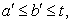then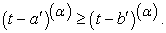2.1. q-Fractional Integral and q-fractional Derivative

Definition 2.2. The Reimann-Liouville q-fractional integral type for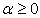is defined by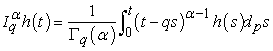(2)

where f is continuous on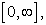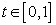and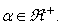And the q-Gamma function is defined as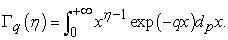Definition 2.3. The Reimann-Liouville q-fractional derivative type foris defined by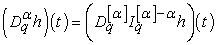(3)

where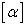is the smallest possible integer greater than or equal to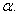Definition 2.4. The Caputo q-fractional derivative type foris defined by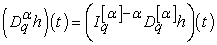(4)

whereis the smallest possible integer greater than or equal toTheorem 2.5. Let t > 0 andThen the following equality holds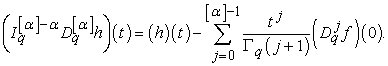(5)

For further details regarding these properties, we refer the reader to 11.

### 3. Mathematical Result

Let us consider a Banach space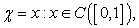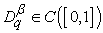given by the norms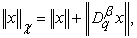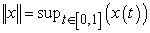and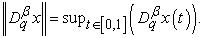Then we have the following Lemmas:

Lemma 3.1. The general solution of q-fractional differential equation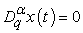foris given by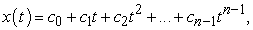(6)

where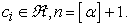Lemma 3.2. Let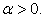Then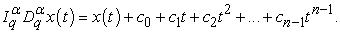Lemma 3.3. Let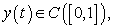then the solution for the equation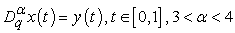(7)

subject to the boundary conditionsis given by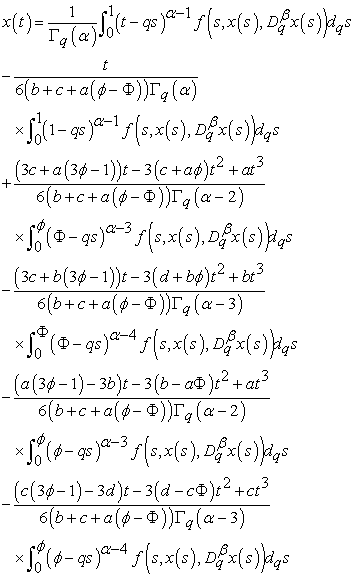(8)

where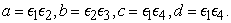Proof. By lemmas 3.1 and 3.2, general solution of (7) is given by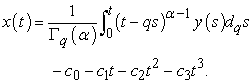(9)

and using the boundary condition, we can get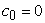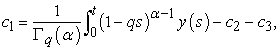and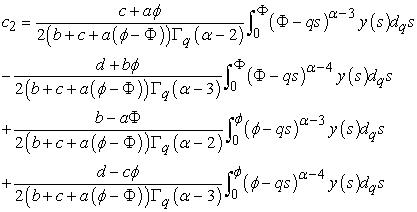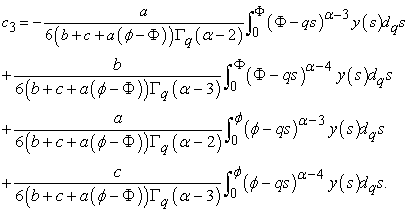Substituting these obtained constant to equation (9) and making use of the lemma (3.3), we can have (8).

3.1. Existence of the Solution

In this section, we will show that there exist a solution of the problem (1) and show the contribution of the term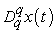on the solution. Suppose the following quantities: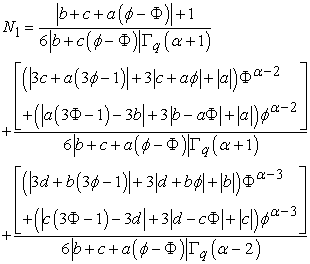(10)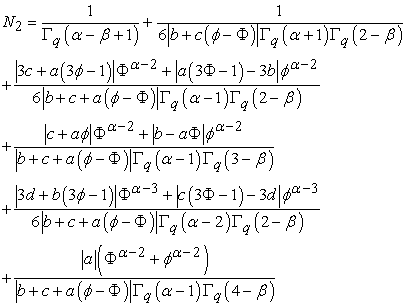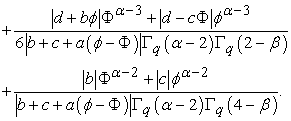(11)

Following the hypotheses of Houas and Dahmani 3, we can consider the following the hypothesis:

Hypothesis 3.4. The function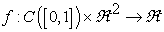is continous.

In accordance also to the work of El-Shahed and Al-Yami 12, we have

Hypothesis 3.5. Assume that there exist a nonegative constant M > 0 such that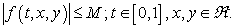Hypothesis 3.6. There exist a nonnegative function u, v on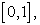such that for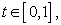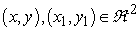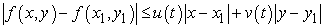where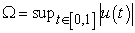and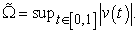Theorem 3.7. Suppose hypothesis (3.6) holds if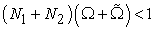then the solution for the problem (1) exist on [0, 1], 1.

Proof. Consider a q-operator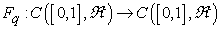such that, it is defined as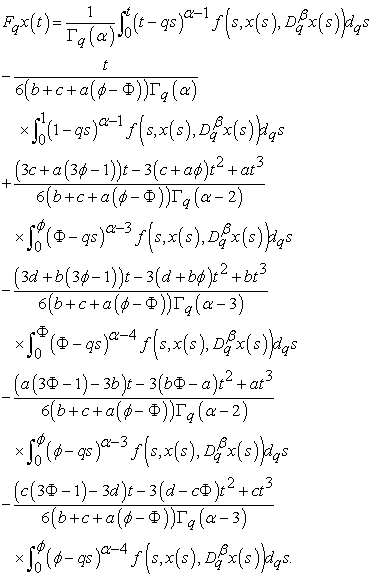In which we must show that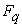is a contraction

For any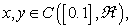using Hypothesis 3.6 we can obtain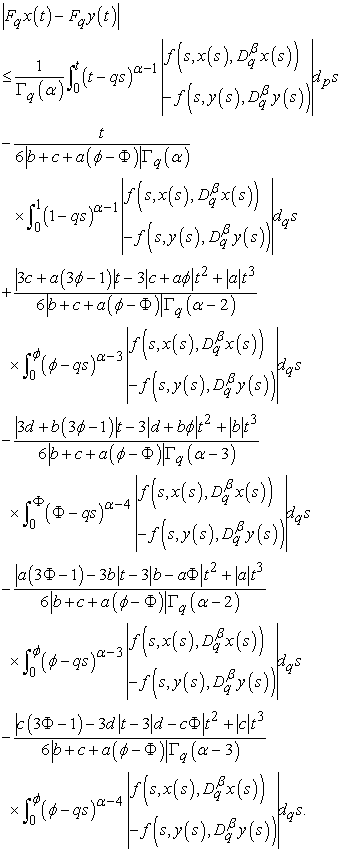To reduce the expression, we use Hypothesis (3.5) and (3.6) and simplifying further we can obtain the relation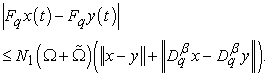Furthermore, to finally show the contraction of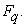We can use the definition of a q-Caputo Fractional Derivative 3, 11, by lemma (3.3) and lastly the hypotheses (3.4-3.6). we can finally obtain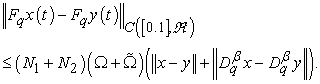### 4. Conclusion

Hence, by the hypothesis (3.6), we deduced thatis a consequence of Banach contraction principle and the solution of equation (1) exist for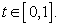For a Generic case, we can say that the contraction obtained in 12, which is a three point q-fractional boundary problem, coincides with the obtained result in this study.

### Acknowledgements

The authors would like to thank the Department of Physics, College of Natural Sciences and Mathematics, Minadanao State University-Main Campus, Marawi City.

### References

  Z. Bai, Y Zhang. Solvability of Fractional Three-Point Boundary Value Problems with Nonlinear growth, Appl. Math. Comp., 218(5), pp. 1719-1725, 2011. In article View Article  M.E Bengrine, Z. Dahmani, Boundary Value Problems for Fractional Differential Equations, Int. J. Open Prob. Comp. Math. 5(4), 2012. In article View Article  M. Houas, Z. Dahmani, New Results for Caputo Boundary Value Problem, Am. J. Comp. App. Math, 3(3), pp. 143-161, 2013. In article View Article  M. Houas, Z. Dahmani, New Results for Differential Equations of Arbitrary Order, IJMMS Journal Int'l Press. In article  A.A. Kilbas, S.A. Marzan, Nonlinear Differential Equation with the Caputo Fractional Derivative in the Space of Continuously differentiable function, Diff. Eq., 41(1), pp. 84-89, 2005. In article View Article  A.M El-Sayed, Nonlinear Functional Differential Equations of Arbitrary Orders, Nonlinear Analysis, 33(2), pp. 181-186, 1998. In article View Article  S. Zhang, S. Chen, J. Lu, Upper and Lower Solution Method for fourth-order Four-point Boundary Value Problems, J. Diff. Eq., 2(36), pp. 12-19, 2006. In article View Article  F. H. Jackson, On q-Functions and Certain Difference Operator, Trans. Roy. Soc., Edinburgh 46, pp. 253-281, 1908. In article View Article  F. H. Jackson, On q-Definite Integrals, Quart. J. Pure Appl. Math, 41, pp.193-203, 1910. In article View Article  V. Kac, P. Cheung, Quantum Calculus, Springer-Verlag, New York, 2002. In article View Article  M. S. Stankovic, et. al, On q-fractional derivatives of Reimann-Liouville and Caputo Type, arXiv:0909, 2009. In article View Article  M. El-Shahed, M. Al-Yami, On the Existence and Uniqueness of Solution for Q-fractional Boundary Value Problem, Int'l J. Math. Anal., vol 5. no. 33, pp. 1619-1630, 2011. In article View ArticleThis work is licensed under a Creative Commons Attribution 4.0 International License. To view a copy of this license, visit http://creativecommons.org/licenses/by/4.0/